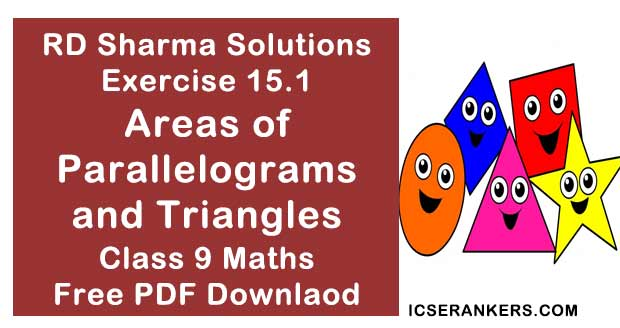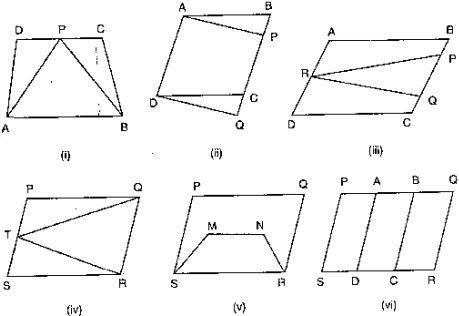# Chapter 15 Areas of Parallelograms and Triangles RD Sharma Solutions Exercise 15.1 Class 9 MathsChapter Name RD Sharma Chapter 15 Areas of Parallelograms and Triangles Exercise 15.1 Book Name RD Sharma Mathematics for Class 10 Other Exercises Exercise 15.2Exercise 15.3 Related Study NCERT Solutions for Class 10 Maths

### Exercise 15.1 Solutions

1. Which of the following figures lie on the same base and between the same parallels. In such a case, write the common base and two parallels.Solution

(i) ΔPCD and trapezium ABCD or on the same base CD and between the same parallels AB and DC.

(ii) Parallelogram ABCD and APQD are on the same base AD and between the same parallels AD and BQ.

(iii) Parallelogram ABCD and ΔPQR are between the same parallels AD and BC but they are not on the same base.

(iv) ΔQRT and parallelogram PQRS are on the same base QR and between the same parallels QR and PS

(v) Parallelogram PQRS and trapezium SMNR on the same base SR but they are not between the same parallels.

(vi) Parallelograms PQRS, AQRD, BCQR and between the same parallels also, parallelograms PQRS, BPSC and APSD are between the same parallels.